The Spotify Playlist Tracking Robot
By Shreshta Obla(sko35) and Ved Kumar(vk262)

## Introduction

In this project, we decided to make a robot that plays your Spotify playlist via an API, while tracking you and using your posture to send commands to the playlist to make remote changes. The first step is user identification. In this step, the robot looks for a face that matches one of the reference images of us partners, while excluding other identified faces. Once it identifies a match, it plays their playlist on the linked Spotify account using the API. Then it enters the tracking phase, where it follows the pants of the target to track their position and maintain a near constant distance away from them. While doing this, it also looks for leg postures that are outside of the default position. When one is found, the API sends a remote command to the Spotify account to do things like pause/play the playlist.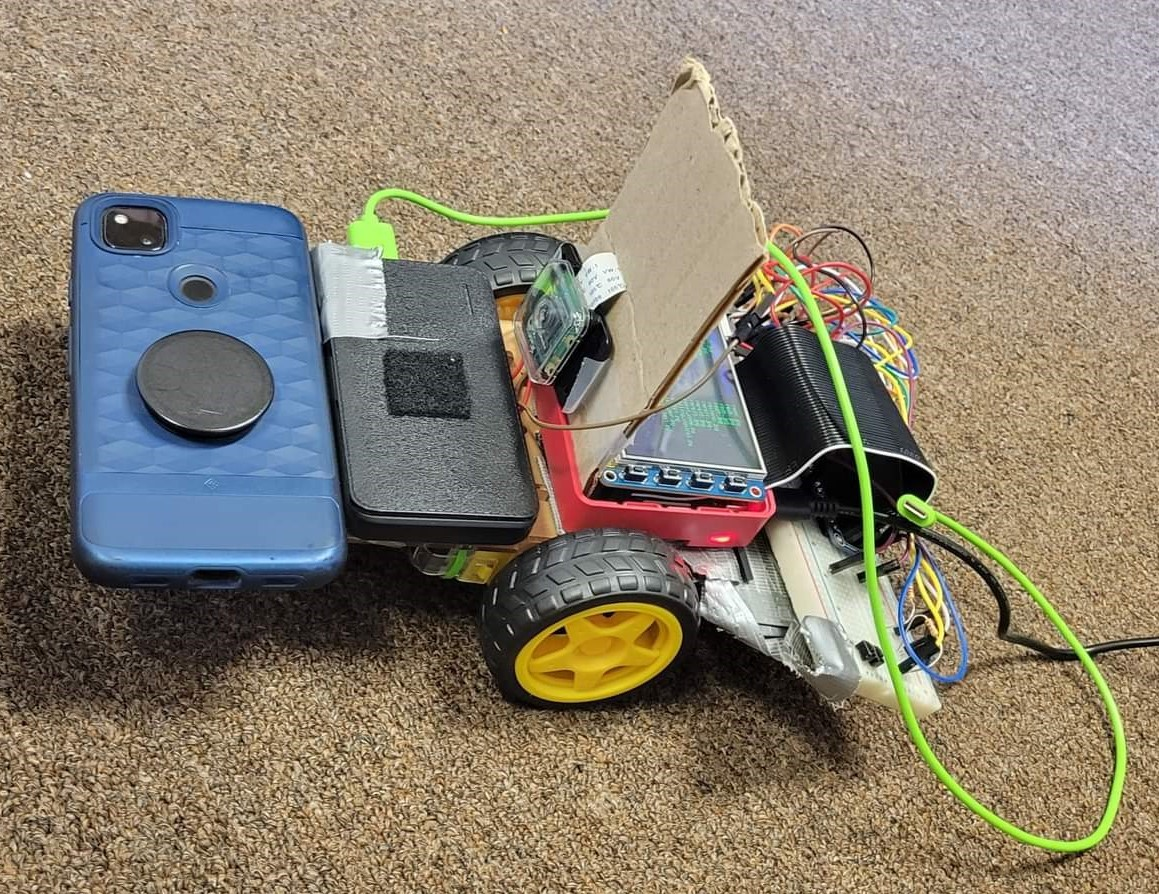## Project Objective:

• Utilize the PiCam to take images and the OpenCV library to filter and process into relevant information
• Use a facial recognition library to identify only one of us partners in images taken by the PiCam
• Setup the Spotify API to work with the correct permissions so all playlists and commands can be forwarded to the Spotify account
• Develop code to process thresholded legs in order to identify the position of the person relative to the robot with respect to distance and angular displacement
• Use positional information of legs to send Spotify commands and move chassis based on displacement to approach optimal position relative to target being followed

## Design

In our first week of work we looked into utilizing the Spotify API for music commands, taking images with the PiCam, and finding a means of facial recognition for identifying both partners for the robot. The PiCam controls were straightforward, and we were able to use Python in taking images with restricted dimensions for optimized processing. We also found a library to use in facial recognition, where it accepts reference images that it does feature extraction on, and with that we were able to develop code that took images, looed for any faces and if they were found they were compared to the reference for a match. On the Spotify side after looking into a lot of the process behind the Spotify API we were able to find some that allowed us to append a playlist to an account queue, pause, and play the playlist. This was made possible by accessing the authorization code of the Spotify account.
Spotify (a python wrapper for Spotify API requests) made authenticating with a Spotify user account easy, given you set user/pass environment variables in Linux. These variables were added to .bashrc, so they would get exported upon startup, and we wouldn’t need to set them up every time. The other task was writing the code to 1. Look up a users playlists, 2. Parse all the tracks, 3. Add them to the play queue, and the pause/play code for gesture control. API requests returned JSON objects that could be parsed easily in python.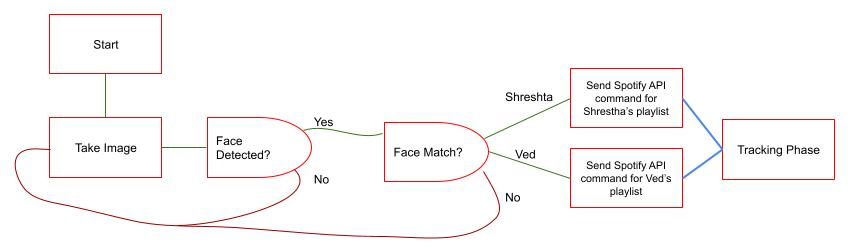#### Figure 1: Facial Recognition Process

For image processing, we referred to some techniques we had learned in ECE5470:Computer Vision, in order to threshold the image and identify relevant information. First, we found HSV thresholds on the image that allowed us to filter out anything except for the pants in the image. This was possible because the color of pants we chose was uncommon in most places, so this filter removed almost all other components of the image leaving us with a binary image of the pants. With this, we used a center of mass algorithm that traveled from left to right until it split the image so that there was a near equal amount of positive pixels on each side of the vertical line. This becomes the horizontal center of mass(COM). With this, the image is then segmented to two halves, where it is assumed that each leg is to contain one leg. Then horizontal COM is repeated for each leg, where their centers are recorded as well. Then a column of the thresholded image is sampled at each of the legs COM horizontally. These columns are used to find the vertical COM at those positions. These become the vertical COM's of the legs. Now we have the coordinates of the rough positions of each leg. Now we use this information to derive the displacement from the target in polar coordinates, meaning angle off of the target and distance away from the target. The angle is found using the proportional distance of the horizontal COM of the pants from the center of the image, which is multiplied by the horizontal FOV of the PiCam, 60 degrees, to find the angle of displacement. For the distance to target, we attempted to use an unusual method of distance calculation.
One of the main aspects of this project was the center-of-mass determination algorithm– This was implemented by scratch through computing the expectation of the normalized pixel intensities, as opposed to using the cv2.moments() function. In hindsight, using this function would have likely saved time, but the experience of implementing the CV aspect + debugging its inner workings was interesting!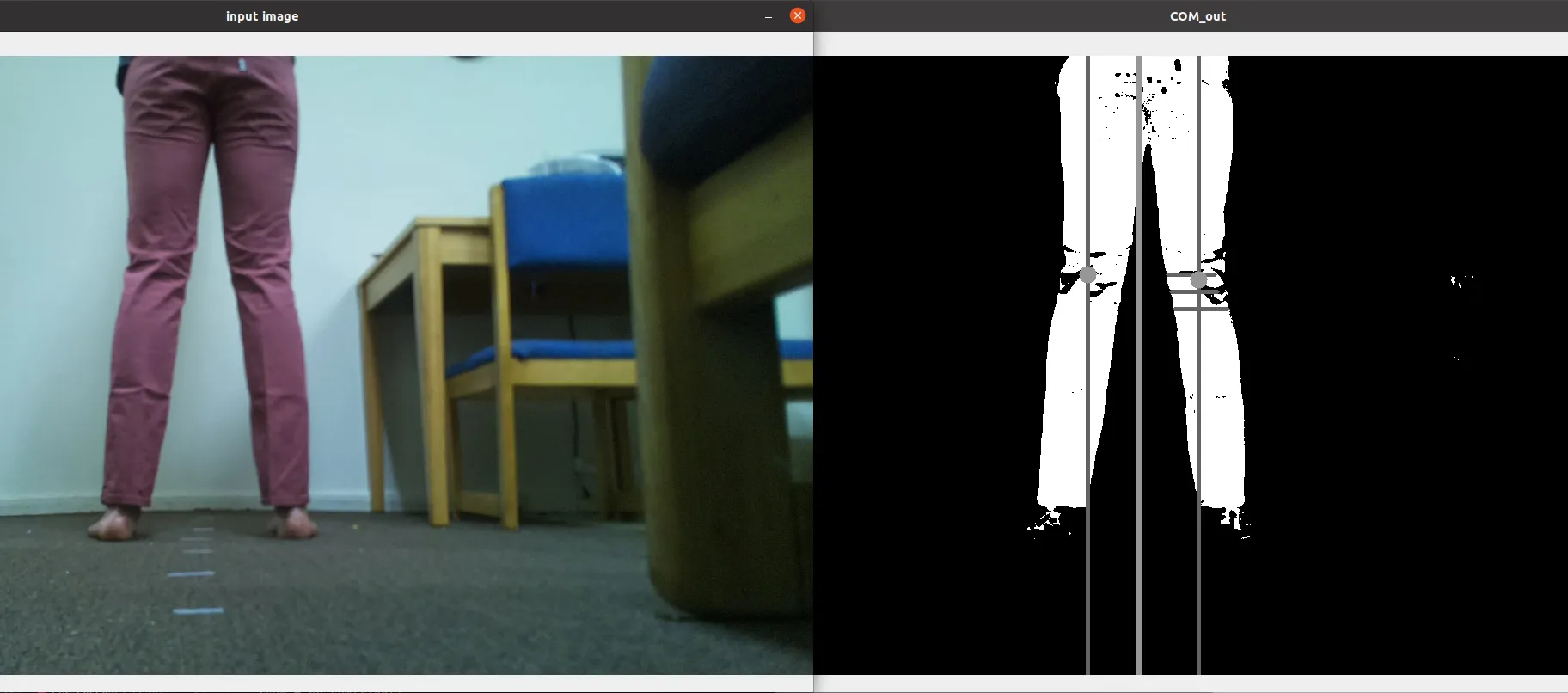#### Figure 2: Image taken (left) with results of thresholding and COM calculations (right)

Our method of distance calculation involved finding the pixel width of the leg and using it's linear relationship to distance to derive a rough estimate of the distance to the target. In order to find the leg width, we worked with the leg that had the lower vertical COM. This is because when a leg is postured, it's elevated above the other, which raises it's COM but also makes it so the leg is at an angle, where a vertical sample of the leg will travel diagonally and cover a much wider set of pixels than what is accurate. With the lower leg, three samples around the vertical COM are averaged together to make the measurement more robust. Once the average width is found, we compare it to a reference width which we provide as an integer. This value was derived from taking an image at a desired optimal distance from the robot to the target, where the leg width was recorded to 61 pixels. This value is subtracted from the sample average, then the results is analyzed. When over zero, the target is closer than in the reference, so the robot must move back. When under zero, the target is too far and the robot must move forward. There is also a buffer range around zero where the target is close enough to the optimal distance where no action is taken. The sign of the difference is used to select the HIGH/LOW assignments to the motors, so that the chassis moves in the correct direction. The difference's magnitude is factor by a coefficient to produce a time that the robot will move for at a fixed speed in order to cover the desired distance. A similar method is used for the angle of displacement to derive how much and in what direction the robot will turn.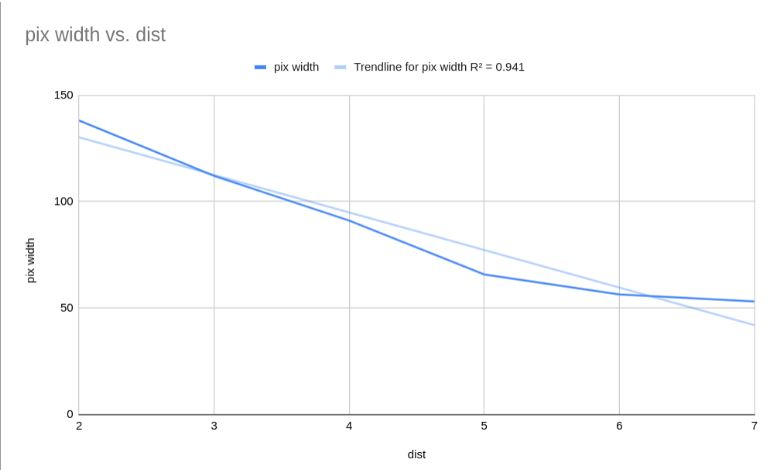#### Figure 3: Linear Distance to Leg Width in Pixels Relation

The next step was to identify postures in thresholded images. We decided to use the angle made between the legs for determining posture as this is resilient to changes in distance and angle of view for the image. When a leg is raised it is identified as a posture of that leg, and when identified it sends a respective command to the Spotify API. In order to find the angle between the legs, we reused the COM coordinates for each leg, while also finding the COM coordinates of the pants themselves to predict where the center of the waist is. With these three points and some standard trigonometry, it is easy to derive the angle of the legs. In order to identify the COM of the waist, we had to use a different strategy than before. We already had the horizontal COM from the pants themselves. To find the vertical position, the thresholded image is observed at only that column on the pants' horizontal COM. The bottom-most pixel is checked first, then moving up one at a time until you reach a positive pixel, meaning you are not looking at the pants. Then, the process keeps going up until it reaches the top of the image or there or the top of the pants. With the vertical start and endpoints the COM coordinates can be derived.
However, this misses a corner case where the waist of the pants isn't in frame, which happens when the camera is too close to the person in some instances. Here, we check that no pants were found by seeing if the y-coordinates are too high on the image, within 30 pixels from the top. Then, we try to estimate the waist's y-position by using the gap between the pants. When the waist is out of frame, the pant legs will never connect, and at the top-most row of the image there will be a number of pixels between where one pant leg ends and the next begins. We use this knowledge and a rough idea of the pant's slope to find the waist's position. The program sweeps the top row looking for pants, when it finds any it goes up to their end and begins counting up until it reaches the other pant leg. This number is factored by a rough slope, 2.5, and made negative to give the y-position of the waist. This is okay as vectors are what is used to find position so negative values are allowable.
Now that we have the three coordinates of the waist, left leg, and right leg; we can make vectors by subtracting the waist coordinates from the legs, giving vectors of the legs. These vectors can be used in a relatively simple trig formula to find the angle between them. Then we check to see if this angle is above a sufficient magnitude, which we chose off of our own flexibility and the flexibility of our pants to be .65 radians. If over this threshold, we then identify which leg has the higher COM y-position. This leg is the one raised, and the respective command is what's sent to the Spotify API. If left, then the playlist is unpaused. If right, then the playlist is paused.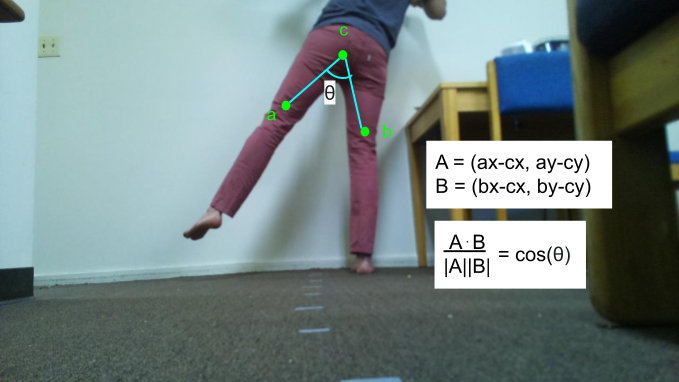#### Figure 4: Angle Derivations Using Leg and Waist Coordinates

In trial however, we found that while meant to be more robust, this method was performing worse, creating more problems than it solved. We decided to try moving towards COM instead, using the difference in y-coordinates to indicate a posture. This method used the difference in the COM of the left and right legs in order to see whether it passed a certain threshold, and if this was met the posture was occuring. Now we tied this logic to the same Spotify commands as we had previously, and used that design instead. While less versatile to distance, since the robot has distance adjustment protocols and only the false negative rate was high, we decided to utilize this for our design.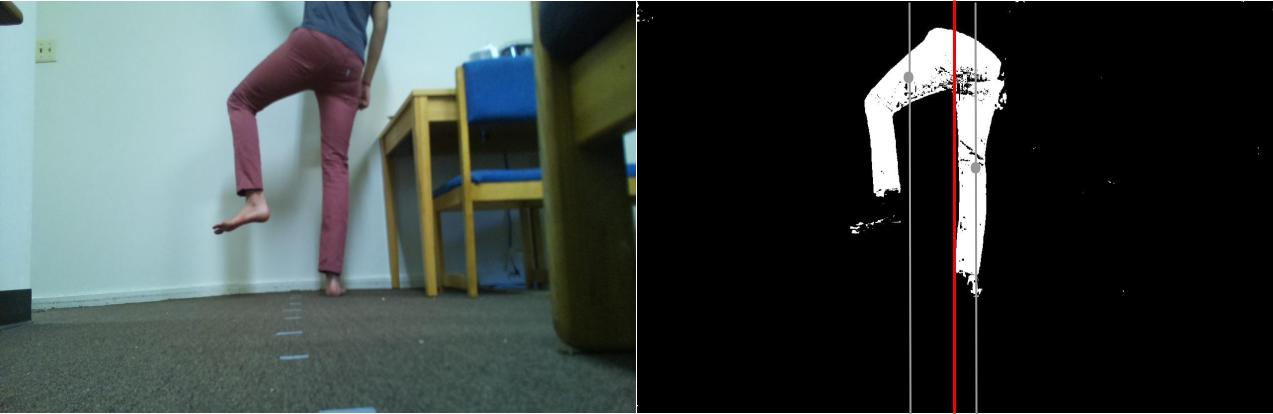#### Figure 5: COM Displacement for Active Posture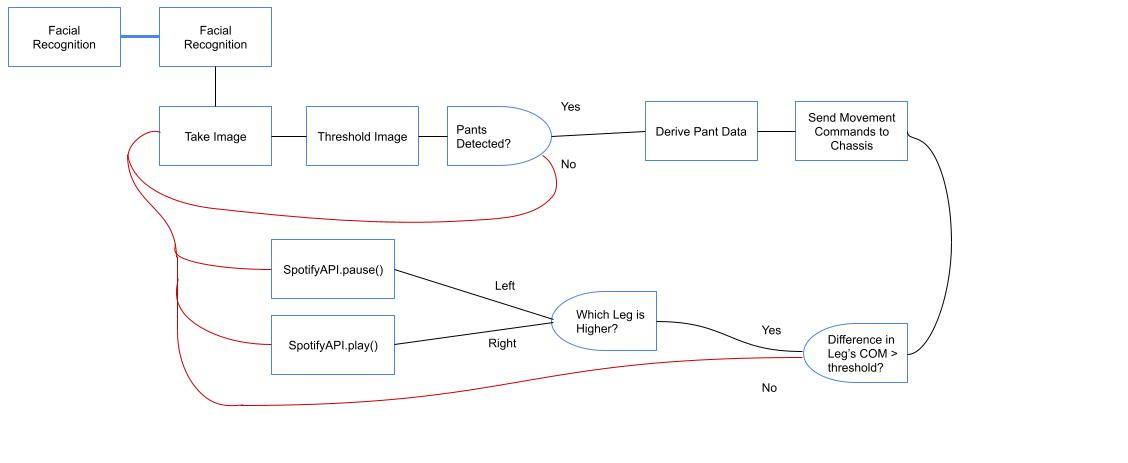## Testing

To test our facial recognition we needed to confirm the two core components of our systems worked properly. One, that a face was found whenever it was in frame, and two, that the faces are correctly discerned as being either Ved, Shreshta, or unknown. To do this, we took reference images of ourselves, and then sample pics that included ourselves, but also empty images as well as image of our roommates. This helped us to debug the case where no faces were found, as our program tried to face match without information which killed the program. We were able to get the face detection working to discern our faces from each other and to keep out others, but there were still some weaknesses. Firstly, an expression on a face made it unidentifiable. Additionally, some faces were incorrectly identified as similar enough to our own that the algorithm would pass them as well. We were unable to get a balanced threshold that blocked them while allowing ourselves every time, but were able to get a general accuracy in performance.
Testing the Spotify API connection was pretty straightforward- we had a player instance running + saw if commands were having desired effects.
For testing the COM algorithm, we took several calibration images (before even getting the robot moving), where the pants were in different positions– up close, few feet away, several feet away, moved to the left + right. To test to see if both the X-center-of-mass (xcm) and Y-center-of-mass (ycm) calculations seemed correct. By testing with several cases, it was easy to pick up on whether the COM was being computed correctly by using our plotting script (thres_test.py), that plots lines at the two legs’ xcms, and places points at their ycms.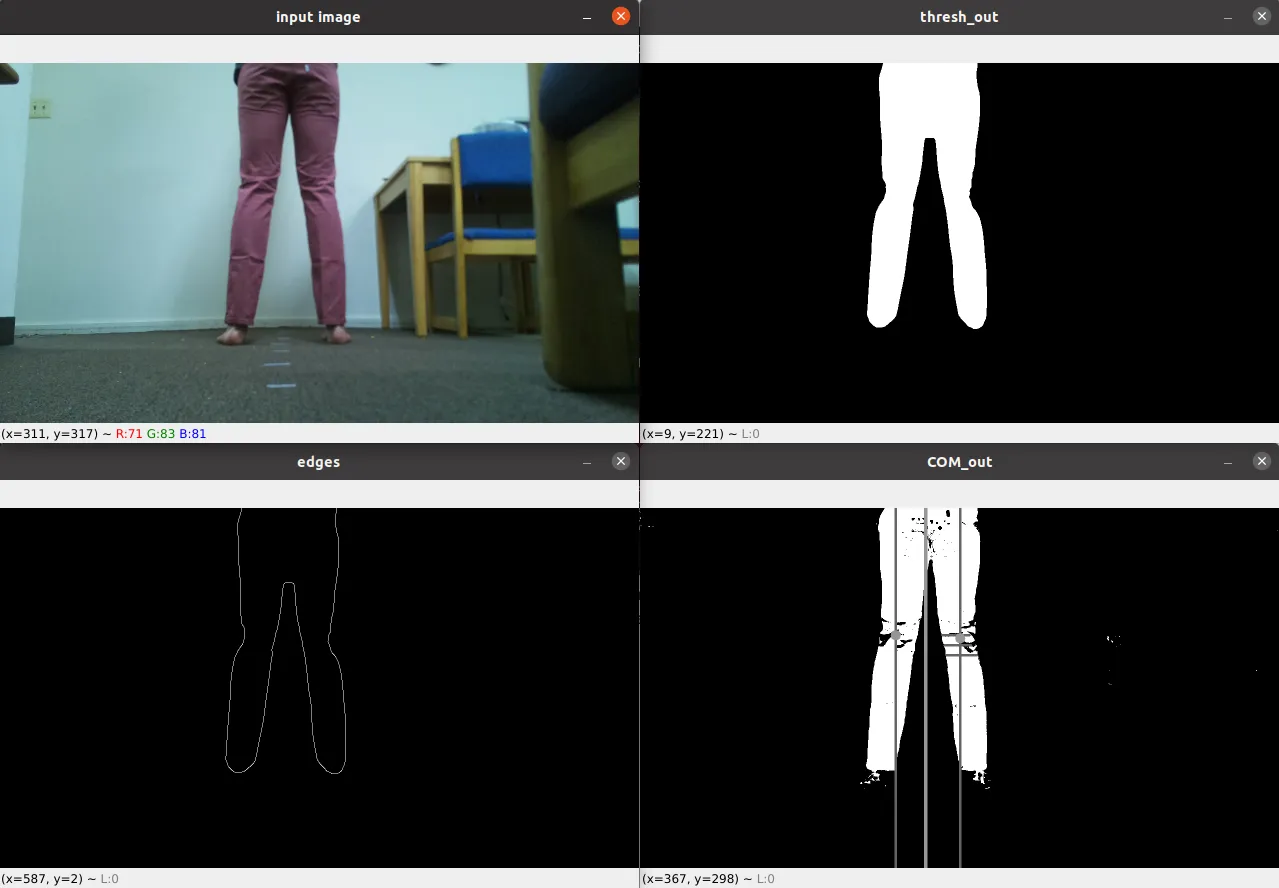#### Figure 7: Image Processing Figures for Testing and Analysis

The computer vision pipeline is depicted above. We tried out several noise filtering methods to reduce noise in the thresholded image. The standard gaussian blur was used to make the intensities more even throughout the image. However, the challenge was to get a good, not ragged edge boundary. (Fig 7) We recalled from our ECE 5470 class that median filters are great for reducing the effects of small splotches of noise. Thus we experimented with the kernel size to ultimately get a smooth boundary whose width could be used for accurate range determination.
For the motor movements we had to spend time into finding the best options for managing motor movements that achieved the desired repositions accurately and without wasting too much time. We used a high duty cycle on the pwm motors, and a frequency of 50. We used measurements of the diameters of the wheels and the diameter of the chassis (distance from wheel to wheel) to calculate a factor from degrees of displacement in degrees to the amount of time to turn for. For forward and backward repositions, we tested a few factors for time from the leg width before we ended up on one we thought was optimal from our estimations. In testing the movements of the robot, we found a problem was in the use of the 3rd wheel having 360 degrees of freedom for swiveling, instead of the standard four-wheel chassis. The issue was that as in shopping carts, after moving a certain direction the wheel would face that direction, and if the robot moved a different way the wheel would resist before giving into the other direction of movement. We suspected that due to the much higher weight of the robot and the carpet being used as terrain rather than tile, this effect was now much more noticeable. This pushed the robot around a lot more than we initially intended for, and we had to account for it in our displacement especially with respect to angular displacement, because now the robot was prone to moving by a lot and losing track of the target without them moving out of frame. To try and help with this, we decided to make the robot adjust angles often in the span of distance movements, so if the robot did spin out it wouldn't cause as much damage as it could.
In testing our posture measurements we used sample images that we knew wouldn't have problems in thresholding or other steps of processing. They consisted of images of normal legs with the waist in frame, images with the waist out of frame and no posture, and images with posture and for some distances. We used these as reference in identifying the factors for how significant of an angle signified a posture. Also, it was here we found the factor to use on the gap between legs in order to roughly estimate the height of the waist through trial and error. Despite the thresholds coming out cleanly we were unable to get a set of parameters that worked in all cases, as it always failed in either posture recognition giving false negatives for false positives with too high of frequency. This forced us to move to COM difference in order to identify postures rather than the angle. With this we tested a few distances to see if the robot false triggered, and found that the robot was quite consistent in only detecting postures on positive cases, although it had a noticeable false negative rate that required a couple of extra iterations to guarantee a posture detection.

## Result

Our goals going into this project were to use facial recognition to identify a registered user, track them while playing their playlist, and take remote commands from them off their posture. As far as the implementation, we developed software using a combination of libraries and our own code to complete everything on this list. However, due to setbacks we didn't predict the performance of the robot for some of these components is lower than we had intended. Because the PiCam adjusts to brightness, and the ample amount of lighting in most areas, the images were prone to inconsistency where they darken to the extent that pants become hard to discern and the threshold fails. Additionally, the shopping cart wheel made otherwise precise movements a lot less controlled, adding a random turn factor to every distance movement. In our initial plan we had said posture detection would be cool to implement but since unrealistic, we would use a microphone for commands as a backup. Luckily though, we were able to get posture detection functional to the point where we didn't feel the need for the microphone, which was great as this was the part of the robot I was most excited to see working alongside everything else. In general, we went into this project with only a few random ideas on how to implement leg detection, and it was fun to use our critical thinking and software design to try to come up with the best way to make a system like this work.

## Conclusions

Looking generally at the process of the project, it was an insight into the reality of project design and execution. In brainstorming we got to consider the possibilities of the RasPi, thinking of ways to apply what we knew with what the RasPi could do, while trying to account for what was achievable within the timeframe we had. In the coming weeks after picking this project we learned a lot about organizing project work through lab reports and segmentation of work. We also came upon problems that blindsided us like the third wheel problem, as we expected no problems from the chassis considering we had already tested it in a previous lab. We were also disappointed by the failure of the angular posture detection, as we were hoping to use that to make a precise posture detection system that may have even been able to handle more than two postures. It was really amazing to see our robot work after having spent so much time on it, as we tested each component of it and they finally all worked and came together into the realization of the idea we had originally made. While the robot fell short in a couple of our goals, we think those largely came down to factors we could have overcome with more time, and even still the work we were able to do was really rewarding and we're proud of the end result.

## Future Work

If we had more time we'd likely invest it into modifying the chassis to have four wheels instead of 3, and see what new options that provided us on movement. We'd also look further into the angle-based posture detection and see if we could get that working, potentially adding new postures to the system. It would also be interesting to change the target identification method to something that is more resilient to brightness, that way our robot could be more consistent and offer even more avenues for exploration.
Additional postures would also allow us to add more cool Spotify API remote commands. One of the big cases that threw off the robot during testing was the case where only one leg was in frame, in which case it should rotate even more to center quickly– addressing this issue would allow our robot to make sharp, responsive turns.

## Work Distribution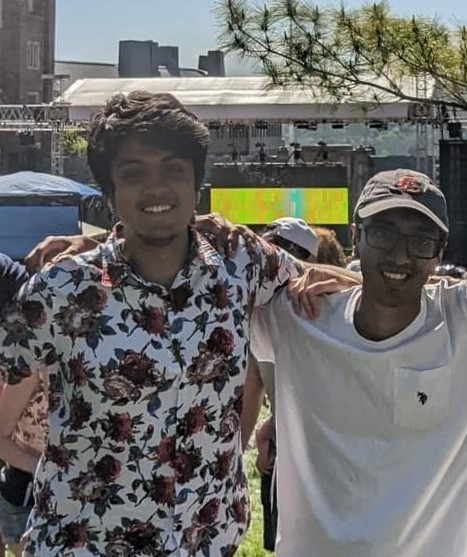#### Project group picture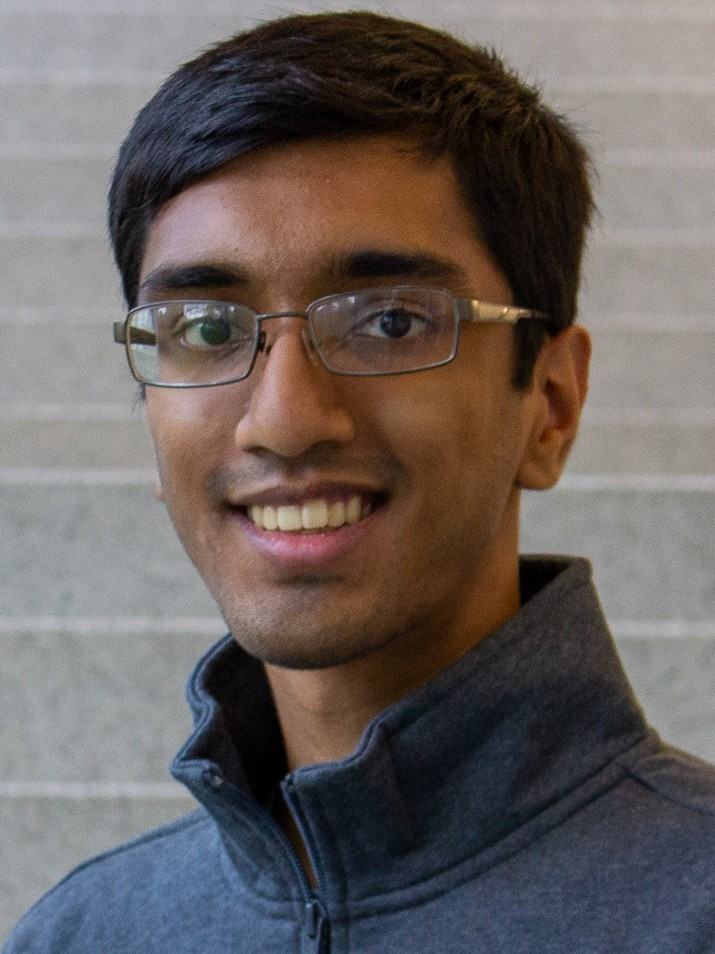### Ved Kumar

vk262@cornell.edu

Implemented image capture and facial recognition, worked on positional updating and posture detection, wrote design and testing report sections on these subjects.### Shreshta Obla

sko35@cornell.edu

Implemented usage systems for Spotify API, developed image processing and center of mass code, and wrote these sections of the report. Made the demonstration video for website.

## Parts List

• Raspberry Pi \$35.00
• Raspberry Pi Camera V2 \$25.00
• Lab 3 robot chassis - Provided in lab

## Code Appendix

Main script

``````
import face_recognition as fr
import spotipy
from spotipy.oauth2 import SpotifyOAuth
import json
import time
import RPi.GPIO as GPIO
import cv2 as cv
import test_scr as tscr
from picamera import PiCamera
from picamera.array import PiRGBArray

GPIO.setmode(GPIO.BCM)

# setup buttons as inputs
GPIO.setup(17, GPIO.IN, pull_up_down=GPIO.PUD_UP)

# set up motor pins for output (left motor)
GPIO.setup(16, GPIO.OUT)
GPIO.setup(5, GPIO.OUT)
GPIO.setup(6, GPIO.OUT)

# set up motor pins for output (right motor)
GPIO.setup(26, GPIO.OUT)
GPIO.setup(20, GPIO.OUT)
GPIO.setup(21, GPIO.OUT)

# initialize motor directions to halt
GPIO.output(20, GPIO.LOW)
GPIO.output(21, GPIO.LOW)

GPIO.output(5, GPIO.LOW)
GPIO.output(6, GPIO.LOW)

# assuming 5/21 high, 6/20 low is CW
# assuming 5/21 low, 6/20 high is CCW
# assuming forward == 5 high, 6 low, 20 low, 21 high
# reverse == 5 low, 6 high, 20 high, 21 low
# 20/21 left wheel, 5/6 right wheel

# init pwm for motors
p = GPIO.PWM(16, 50)
p2 = GPIO.PWM(26, 50)

p.start(99)
p2.start(99)

# spotify authentication env vars to set up:
# export SPOTIPY_CLIENT_ID='110cd74a32b94b6e91cbeb131871ebd4'
# export SPOTIPY_CLIENT_SECRET='46f0108512844f098145e90fdb951dda'
# export SPOTIPY_REDIRECT_URI='http://127.0.0.1:9090'
# all from account!

# authenticate
sp = spotipy.Spotify(auth_manager=SpotifyOAuth(scope=scope))

vk_enc = fr.face_encodings(vk)
s_enc = fr.face_encodings(s)

cam = PiCamera()
cam.rotation = 180
cam.resolution = (1280, 720)
rawCapture = PiRGBArray(cam)
rawCapture2 = PiRGBArray(cam)

match = 0
for image in cam.capture_continuous(rawCapture, format="bgr", use_video_port=True):

#while match == 0:
print("iter")
x = 0
#cam.capture(rawCapture, format="bgr", use_video_port = True)
image = rawCapture.array
cv.imwrite("test.jpg",image)

floc = fr.face_locations(test)
if len(floc) != 0:
test_enc = fr.face_encodings(test)

vedM = fr.face_distance([vk_enc],test_enc)
sM = fr.face_distance([s_enc],test_enc)
if vedM <= sM:
r = fr.compare_faces([vk_enc], test_enc)
if r:
match = 1
print("ved")
tscr.play_veds(sp)

# get veds playlists
# ved_playlists = sp.user_playlists('mixtapeman789', limit=50, offset=0 )

# ved_songs = []

# for idx, item in enumerate(ved_playlists['items']):
#     playlist = item['uri']
#     tracks = sp.playlist_tracks(playlist)
#     # print(tracks['items'])
#     print("NEW PLAYLIST \n")

#     for idx2, item2 in enumerate(tracks['items']):
#         print(item2['track']['uri'])
#         ved_songs.append(item2['track']['uri'])

# sp.start_playback(uris=ved_songs)

break
else:
r = fr.compare_faces([s_enc],test_enc)
if r:
match = 2
print("shreshta")
tscr.play_shreshtas(sp)

# # get shreshtas playlists
# shreshta_playlists = sp.current_user_playlists(limit=10)
# shreshta_songs = []

# for idx, item in enumerate(shreshta_playlists['items']):
#     playlist = item['uri']
#     tracks = sp.playlist_tracks(playlist)
#     # print(tracks['items'])
#     print("NEW PLAYLIST \n")

#     for idx2, item2 in enumerate(tracks['items']):
#         print(item2['track']['uri'])
#         shreshta_songs.append(item2['track']['uri'])

# sp.start_playback(uris=shreshta_songs)
break

rawCapture.truncate(0)

print("5 seconds before following begins")
time.sleep(5)

img_idx = 0
t0 = time.time()

paused = False
for image in cam.capture_continuous(rawCapture2, format="bgr", use_video_port=True):
print("we working??")

if ( time.time() >= t0+180 or (not GPIO.input(17)) ):
break
else:

# assuming image is an RGB numpy array (equivalent to doing cv.imread())

image = rawCapture2.array

#image = image[...,::-1].copy()

cv.imwrite(str(img_idx) + ".jpg", image)

state_var, x_dist, y_dist = tscr.update_COM(image)

if ( state_var == 1 ):
# there's no output if it succeeds?
print("left")
#if ( (sp.current_playback())["is_playing"] == "true" ): ### condition on whether currently playing )
if ( paused == False):
sp.pause_playback()
paused = True
#else:
#sp.start_playback()

#  elif ( state_var == 2 ):
#     sp.previous_track()

elif ( state_var == 3 ):
print("right")
if (paused == True):
sp.start_playback()
paused = False
#sp.next_track()

# elif ( state_var == 4 ):

# x_dist -> motor output
if ( x_dist > 0 ):
GPIO.output(20, GPIO.HIGH)
GPIO.output(21, GPIO.LOW)
GPIO.output(5, GPIO.LOW)
GPIO.output(6, GPIO.HIGH)
else:
GPIO.output(5, GPIO.HIGH)
GPIO.output(6, GPIO.LOW)
GPIO.output(21, GPIO.HIGH)
GPIO.output(20, GPIO.LOW)

# linear function based on x_dist:
x_rot_time = 0.0006*abs(x_dist)
print("start_x_pause" )
time.sleep(x_rot_time)
print(x_rot_time)
print("fin x_pause")
GPIO.output(20, GPIO.LOW)
GPIO.output(5, GPIO.LOW)
GPIO.output(21, GPIO.LOW)
GPIO.output(6, GPIO.LOW)

time.sleep(1)

# y_dist -> motor output
y_rot_time = 0.05*(y_dist-71)
print(y_rot_time)
#time.sleep(10*abs(y_rot_time))

if y_rot_time < -.2 and y_rot_time > -3:
GPIO.output(5, GPIO.HIGH)
GPIO.output(6, GPIO.LOW)
GPIO.output(20, GPIO.HIGH)
GPIO.output(21, GPIO.LOW)
elif y_rot_time > .2 and y_rot_time < 3:
GPIO.output(6, GPIO.HIGH)
GPIO.output(5, GPIO.LOW)
GPIO.output(21, GPIO.HIGH)
GPIO.output(20, GPIO.LOW)
time.sleep(abs(y_rot_time))

GPIO.output(5, GPIO.LOW)
GPIO.output(20, GPIO.LOW)
GPIO.output(21, GPIO.LOW)
GPIO.output(6, GPIO.LOW)

time.sleep(1)
# main loop stuff

# take in the input image
# analyze image to determine distance away from legs
# detemine COM horizontal displacement
# motors update accordingly after parsing (linear equation scale)

# make spots to add functionality for gestures

rawCapture2.truncate(0)

cam.close()
``````

Image Processing Subprogram

``````
import sys
import cv2 as cv
import numpy as np
from matplotlib import pyplot as plt
import spotipy
from spotipy.oauth2 import SpotifyOAuth
import json

print("INSIDE TEST_SCR")
print("INSIDE TEST_SCR")
print("INSIDE TEST_SCR")
print("INSIDE TEST_SCR")
print("INSIDE TEST_SCR")
print("INSIDE TEST_SCR")
print("INSIDE TEST_SCR")
print("INSIDE TEST_SCR")
print("INSIDE TEST_SCR")

def play_shreshtas( sp ):
# get shreshtas playlists
shreshta_playlists = sp.current_user_playlists(limit=10)
shreshta_songs = []

for idx, item in enumerate(shreshta_playlists['items']):
playlist = item['uri']
tracks = sp.playlist_tracks(playlist)
# print(tracks['items'])
print("NEW PLAYLIST \n")

for idx2, item2 in enumerate(tracks['items']):
print(item2['track']['uri'])
shreshta_songs.append(item2['track']['uri'])

sp.start_playback(uris=shreshta_songs)

def play_veds( sp ):
# get veds playlists
ved_playlists = sp.user_playlists('mixtapeman789', limit=50, offset=0 )

ved_songs = []

for idx, item in enumerate(ved_playlists['items']):
playlist = item['uri']
tracks = sp.playlist_tracks(playlist)
# print(tracks['items'])
print("NEW PLAYLIST \n")

for idx2, item2 in enumerate(tracks['items']):
print(item2['track']['uri'])
ved_songs.append(item2['track']['uri'])

sp.start_playback(uris=ved_songs)

def update_COM( img ):

hsv_img = cv.cvtColor(img,cv.COLOR_BGR2HSV)

color_thresh = cv.inRange(hsv_img, np.array([0,117,25]), np.array([10,255,211]))
cv.imwrite('0color_thresh.jpg', color_thresh)

thres_bl = cv.medianBlur(color_thresh, 41 )
edges = cv.Canny(thres_bl, 100,200)

cv.imwrite('0thresh_out.jpg', thres_bl)
cv.imwrite('0edges.jpg', edges)

print('shape0: ' + str(img.shape))
print('shape1: ' + str(img.shape))

# swapped from thres_bl
col = np.sum(color_thresh, axis=0)

# loop across cols in the image until intensities on both sides are ==
mindist = 1000000000000000
min_idx = 0
for x in range(img.shape):
left = col[0:x]
lsum = np.sum(left)
right = col[x:]
rsum = np.sum(right)

if abs(lsum-rsum) <= mindist:
min_idx = x
mindist = abs(lsum-rsum)

# left_x = min_idx
# right_x = max_size - min_idx
left_sum = np.sum(col[0:min_idx])
print(left_sum)
right_sum = np.sum(col[min_idx:])
print(right_sum)

# left_sum + right_sum

# normalize thresholded image + sum columns to get marginal PMF sum
# swapped from thres_bl
left_norm = (np.sum(color_thresh/left_sum, axis=0))
# swapped from thres_bl
right_norm = (np.sum(color_thresh/right_sum, axis=0))

# check to see if partition is working correctly
print("min_idx " + str(min_idx))

# compute expectation of marginal PMFs:
left_xcm = int(np.sum([i*left_norm[i] for i in range(min_idx)]))
right_xcm = int(np.sum([i*right_norm[i] for i in range(min_idx,len(col))]))

print("left_xcm " + str(left_xcm))
print("right_xcm: " + str(right_xcm))

# then find y_cm on top of the selected x_cm's
# swapped from thres_bl
a = np.array(color_thresh)

l_ycm_col = a[:,left_xcm]
r_ycm_col = a[:,right_xcm]

l_sum = np.sum(l_ycm_col)
r_sum = np.sum(r_ycm_col)

print("l_ycm_col: " + str(l_ycm_col) + ", l_sum: " + str(l_sum))
print("r_ycm_col: " + str(r_ycm_col) + ", r_sum: " + str(r_sum))

boll = False

# # normalized columns, find center of mass using index
try:
left_ycm = int(np.sum([i*(l_ycm_col/l_sum)[i] for i in range(img.shape)]))
right_ycm = int(np.sum([i*(r_ycm_col/r_sum)[i] for i in range(img.shape)]))
except ValueError:
print("pants not detected (ycm not computed correctly), goodbye :(")
boll = True

if (boll == False):
print(left_ycm)
print(right_ycm)

print(len(edges[left_ycm]))

ycm = []

if ( left_ycm > right_ycm ):
ycm = [left_ycm, left_ycm+20, left_ycm+40]

else:
ycm = [right_ycm, right_ycm+20, right_ycm+40]

edge_idx_1 = [0,0,0]
edge_idx_2 = [0,0,0]

if ( ycm == left_ycm ):
print("left leg posture detect")
# loop through image to find leg thickness
for y in range(len(ycm)):
for x in range(len(edges[ycm[y]])):
val = edges[ycm[y]][x]
if ( val != 0 and edge_idx_1[y] == 0 ):
print(x)
edge_idx_1[y] = x
elif ( val != 0 and edge_idx_1[y] !=0 ):
print(x)
edge_idx_2[y] = x
break

else:
print("right leg posture detect")
# loop through image to find leg thickness
for y in range(len(ycm)):
for x in range( (len(edges[ycm[y]])-1), -1,-1 ):
val = edges[ycm[y]][x]
if ( val != 0 and edge_idx_1[y] == 0 ):
print(x)
edge_idx_1[y] = x
elif ( val != 0 and edge_idx_1[y] !=0 ):
print(x)
edge_idx_2[y] = x
break

print(edge_idx_1)
print(edge_idx_2)

# determine vertical distance parameter:
y_dist_sum = 0
for i in range(len(ycm)):
y_dist_sum += abs(edge_idx_2[i]-edge_idx_1[i])

y_avg_dist = y_dist_sum/3.0
print("y_avg_dist: " + str(y_avg_dist))

if (boll == True):
y_avg_dist = 71
print("we printing correctly")

# determine horizontal distance parameter:
# negative -> min_idx partition to the left of center, need to rotate left.
x_dist = min_idx - img.shape/2
print("x_dist: " + str(x_dist))

if (boll == True):
x_dist = 0

state_var = 0
# determine any gestures + accordingly set state variable:
# 1. leg halfway up
if not boll:
ydif = left_ycm - right_ycm
if ydif > 50:
state_var = 1
elif ydif < -50:
state_var = 3

# top central COM point, loop through rows, find first 255 location
# find angle between this point and y_cm on posture leg

# 2. leg fully up

return state_var, x_dist, y_avg_dist

``````

Image Processing Testing and Visualization

``````
import cv2 as cv
import numpy as np
from matplotlib import pyplot as plt

hsv_img = cv.cvtColor(img,cv.COLOR_BGR2HSV)

color_thresh = cv.inRange(hsv_img, np.array([119,0,0]), np.array([179,115,140]))
cv.imshow('color_thresh', color_thresh)

# ret,thres = cv.threshold(thresh_img, 45,255,cv.THRESH_BINARY_INV)
thres_bl = cv.GaussianBlur(color_thresh, (13,13), 0)

cv.imshow('thresh_out', thres_bl)
cv.waitKey(0)

print('shape0: ' + str(img.shape))
print('shape1: ' + str(img.shape))

col = np.sum(thres_bl, axis=0)

mindist = 1000000000000000
min_idx = 0
for x in range(img.shape):
#    for y in range(len(img.shape)):
left = col[0:x]
# print(left)
lsum = np.sum(left)
right = col[x:]
rsum = np.sum(right)
# print(abs(lsum-rsum))

if abs(lsum-rsum) <= mindist:
min_idx = x
mindist = abs(lsum-rsum)

# for x in range()

cv.line(color_thresh, (min_idx,0), (min_idx, 2464), 150, 5)

# 2465 pixels down
# min_idx is partition (2465 * min_idx pixels)

# left_x = min_idx
# right_x = max_size - min_idx

left_sum = np.sum(col[0:min_idx])
print(left_sum)
right_sum = np.sum(col[min_idx:])
print(right_sum)

# left_sum + right_sum

# np.sum()
left_norm = (np.sum(thres_bl/left_sum, axis=0))
right_norm = (np.sum(thres_bl/right_sum, axis=0))

# # marg = np.sum(normalized, axis=0)
# left_marg = (np.sum(left_norm, axis=0))[0:min_idx]
# right_marg = (np.sum(normalized, axis=0))[min_idx:]

# check to see if partition is working correctly
print("min_idx " + str(min_idx))
# print("marg len " + str(len(marg)))
# print("right_marg len " + str(len(right_marg)))

# print([i*marg[i] for i in range(min_idx)])
left_xcm = int(np.sum([i*left_norm[i] for i in range(min_idx)]))
right_xcm = int(np.sum([i*right_norm[i] for i in range(min_idx,len(col))]))

# then find y_cm on top of the selected x_cm's

print("left_xcm " + str(left_xcm))
print("right_xcm: " + str(right_xcm))

cv.line(color_thresh, (left_xcm,0), (left_xcm, 2464), 100, 4)
cv.line(color_thresh, (right_xcm,0), (right_xcm, 2464), 100, 4)

a = np.array(thres_bl)
l_ycm_col = a[:,left_xcm]
r_ycm_col = a[:,right_xcm]

print(l_ycm_col)
l_sum = np.sum(l_ycm_col)
r_sum = np.sum(r_ycm_col)

# # normalized columns, find center of mass using index
# l_norm = l_ycm_col/l_sum
# r_norm = r_ycm_col/r_sum

left_ycm = int(np.sum([i*(l_ycm_col/l_sum)[i] for i in range(img.shape)]))
right_ycm = int(np.sum([i*(r_ycm_col/r_sum)[i] for i in range(img.shape)]))

print(left_ycm)
print(right_ycm)

cv.circle(color_thresh, (left_xcm, left_ycm), radius=10, color=150, thickness=-1)
cv.circle(color_thresh, (right_xcm, right_ycm), radius=10, color=150, thickness=-1)

plt.imshow(color_thresh,'gray')
# plt.imshow(imgg, 'gray')
plt.show()
``````

Image Processing Program Using Angles, not effective

``````
import sys
import cv2 as cv
import numpy as np
from matplotlib import pyplot as plt
import spotipy
from spotipy.oauth2 import SpotifyOAuth
import json
import math

print("INSIDE TEST_SCR")

def play_shreshtas( sp ):
# get shreshtas playlists
shreshta_playlists = sp.current_user_playlists(limit=10)
shreshta_songs = []

for idx, item in enumerate(shreshta_playlists['items']):
playlist = item['uri']
tracks = sp.playlist_tracks(playlist)
# print(tracks['items'])
print("NEW PLAYLIST \n")

for idx2, item2 in enumerate(tracks['items']):
print(item2['track']['uri'])
shreshta_songs.append(item2['track']['uri'])

sp.start_playback(uris=shreshta_songs)

def play_veds( sp ):
# get veds playlists
ved_playlists = sp.user_playlists('mixtapeman789', limit=50, offset=0 )

ved_songs = []

for idx, item in enumerate(ved_playlists['items']):
playlist = item['uri']
tracks = sp.playlist_tracks(playlist)
# print(tracks['items'])
print("NEW PLAYLIST \n")

for idx2, item2 in enumerate(tracks['items']):
print(item2['track']['uri'])
ved_songs.append(item2['track']['uri'])

sp.start_playback(uris=ved_songs)

def update_COM( img ):

hsv_img = cv.cvtColor(img,cv.COLOR_BGR2HSV)

#color_thresh = cv.inRange(hsv_img, np.array([0,117,25]), np.array([10,255,211]))
color_thresh = cv.inRange(hsv_img, np.array([119,1,2]), np.array([179,115,141]))
cv.imwrite('0color_thresh.jpg', color_thresh)

thres_bl = cv.medianBlur(color_thresh, 41 )
edges = cv.Canny(thres_bl, 100,200)

cv.imwrite('0thresh_out.jpg', thres_bl)
cv.imwrite('0edges.jpg', edges)

print('shape0: ' + str(img.shape))
print('shape1: ' + str(img.shape))

# swapped from thres_bl
col = np.sum(color_thresh, axis=0)

# loop across cols in the image until intensities on both sides are ==
mindist = 1000000000000000
min_idx = 0
for x in range(img.shape):
left = col[0:x]
lsum = np.sum(left)
right = col[x:]
rsum = np.sum(right)

if abs(lsum-rsum) <= mindist:
min_idx = x
mindist = abs(lsum-rsum)

# left_x = min_idx
# right_x = max_size - min_idx
left_sum = np.sum(col[0:min_idx])
print(left_sum)
right_sum = np.sum(col[min_idx:])
print(right_sum)

# left_sum + right_sum

# normalize thresholded image + sum columns to get marginal PMF sum
# swapped from thres_bl
left_norm = (np.sum(color_thresh/left_sum, axis=0))
# swapped from thres_bl
right_norm = (np.sum(color_thresh/right_sum, axis=0))

# check to see if partition is working correctly
print("min_idx " + str(min_idx))

# compute expectation of marginal PMFs:
left_xcm = int(np.sum([i*left_norm[i] for i in range(min_idx)]))
right_xcm = int(np.sum([i*right_norm[i] for i in range(min_idx,len(col))]))

print("left_xcm " + str(left_xcm))
print("right_xcm: " + str(right_xcm))

# then find y_cm on top of the selected x_cm's
# swapped from thres_bl
a = np.array(color_thresh)

l_ycm_col = a[:,left_xcm]
r_ycm_col = a[:,right_xcm]

l_sum = np.sum(l_ycm_col)
r_sum = np.sum(r_ycm_col)

print("l_ycm_col: " + str(l_ycm_col) + ", l_sum: " + str(l_sum))
print("r_ycm_col: " + str(r_ycm_col) + ", r_sum: " + str(r_sum))

boll = False

# # normalized columns, find center of mass using index
try:
left_ycm = int(np.sum([i*(l_ycm_col/l_sum)[i] for i in range(img.shape)]))
right_ycm = int(np.sum([i*(r_ycm_col/r_sum)[i] for i in range(img.shape)]))
except ValueError:
print("pants not detected (ycm not computed correctly), goodbye :(")
boll = True

if (boll == False):
print(left_ycm)
print(right_ycm)

print(len(edges[left_ycm]))

ycm = []

if ( left_ycm > right_ycm ):
ycm = [left_ycm, left_ycm+20, left_ycm+40]

else:
ycm = [right_ycm, right_ycm+20, right_ycm+40]

edge_idx_1 = [0,0,0]
edge_idx_2 = [0,0,0]

if ( ycm == left_ycm ):
print("left leg posture detect")
# loop through image to find leg thickness
for y in range(len(ycm)):
for x in range(len(edges[ycm[y]])):
val = edges[ycm[y]][x]
if ( val != 0 and edge_idx_1[y] == 0 ):
print(x)
edge_idx_1[y] = x
elif ( val != 0 and edge_idx_1[y] !=0 ):
print(x)
edge_idx_2[y] = x
break

else:
print("right leg posture detect")
# loop through image to find leg thickness
for y in range(len(ycm)):
for x in range( (len(edges[ycm[y]])-1), -1,-1 ):
val = edges[ycm[y]][x]
if ( val != 0 and edge_idx_1[y] == 0 ):
print(x)
edge_idx_1[y] = x
elif ( val != 0 and edge_idx_1[y] !=0 ):
print(x)
edge_idx_2[y] = x
break

print(edge_idx_1)
print(edge_idx_2)

# determine vertical distance parameter:
y_dist_sum = 0
for i in range(len(ycm)):
y_dist_sum += abs(edge_idx_2[i]-edge_idx_1[i])

y_avg_dist = y_dist_sum/3.0
print("y_avg_dist: " + str(y_avg_dist))

if (boll == True):
y_avg_dist = 71
print("we printing correctly")

# determine horizontal distance parameter:
# negative -> min_idx partition to the left of center, need to rotate left.
x_dist = min_idx - img.shape/2
print("x_dist: " + str(x_dist))

if boll:
x_dist = 0

if not boll:
# min_idx = xcm
y_cm = 720
state_var = 0
nfound = True
leg = False
while nfound and y_cm > 0:
y_cm -= 1
if img[y_cm][min_idx] > 200:
leg = True
if leg:
if img[y_cm][min_idx] < 30:
nfound = False

leg = False
lFound = True
gapC = False
xpos = 0
count = 0
if y_cm < 30:
while lFound and xpos < 1279:
xpos += 1
if img[xpos]>200:
leg = True
if gapC:
lFound = False
else:
if leg:
leg = False
if leg:
count+=1
if not leg and not gapC and count > 0:
if count > 30:
gapC = True
count = 0
if gapC:
count+=1

print(count)
if count < 100:
y_cm = y_cm - count*2.5

ax = left_xcm - min_idx
ay = left_ycm - y_cm
bx = right_xcm - min_idx
by = right_ycm - y_cm

angle = math.acos(float(ax*bx+ay*by)/( (float(ax**2+ay**2)**(0.5)) * (float(bx**2+by**2)**(0.5)) ))
#  determine any gestures + accordingly set state variable:
# 1. leg halfway up
if angle > .6:
if left_ycm > right_ycm:
state_var = 1
else:
state_var = 3
#print("Dot: " + str(float(ax*bx+ay*by)) + "   magA:  "+ str (float(ax**2+ay**2)**(0.5)) + " magB:    " + str(float(bx**2+by**2)**(0.5)))
print([(min_idx,y_cm), (left_xcm, left_ycm), (right_xcm, right_ycm)])

# top central COM point, loop through rows, find first 255 location
# find angle between this point and y_cm on posture leg

cv.circle(color_thresh, (left_xcm, left_ycm), radius=10, color=150, thickness=-1)
cv.circle(color_thresh, (right_xcm, right_ycm), radius=10, color=150, thickness=-1)
cv.imwrite("0com.jpg", color_thresh)

# 2. leg fully up

return state_var, x_dist, y_avg_dist
``````